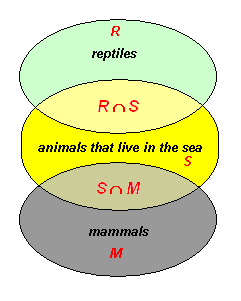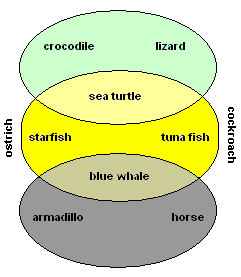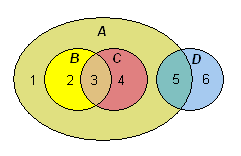# Venn Diagrams

Venn diagrams are a way to graphically represent sets , subsets , intersections , and unions . They are named after John Venn, who started using them in $1880$ .

Suppose $R$ is the set of all reptiles, $S$ is the set of all creatures that live in the sea, and $M$ is the set of all mammals. We get the Venn diagram:The region labelled $R\cap S$ is the intersection of $R$ and $S$ ; the set of reptiles which live in the sea. Similarly $S\cap M$ is the set of mammals that live in the sea. Since there is no such thing as an animal which is both a reptile and a mammal, the intersection $R\cap M$ is empty (the $R$ and $M$ regions don't cross over each other).

Below we show some examples of animals in each category of the Venn diagram.For another example, let $A=\left\{1,2,3,4,5\right\}$ , $B=\left\{2,3\right\}$ , $C=\left\{3,4\right\}$ , $D=\left\{5,6\right\}$ . A Venn diagram for this situation would look like this: